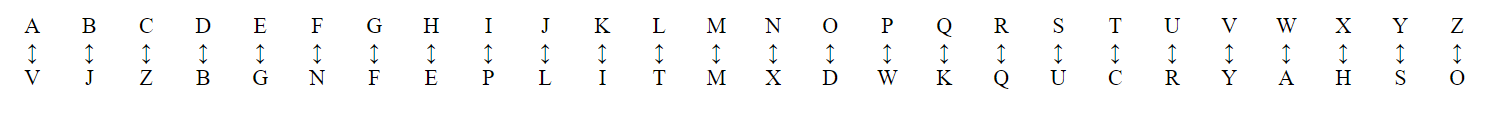# Decrypting a Substitution Cipher¶

Total points possible Uses
10 LED display

## Description¶

The substitution cipher is deceptively easy. Messages are encrypted using a key which is created in advance. You make the key by jumbling up the alphabet like this:Your goal is to turn your micro:bit into a machine that can decode messages using a substitution cipher. We call the message that has been encrypted cipher text and the decrypted message plain text. You will need to store the alphabet with the substition cipher in your program. You can use a python dictionary to do this. A python dictionary for the substitution cipher above looks like this:

cipher_key = { 'A':'V', 'B':'J', 'C':'Z', 'D':'B', 'E':'G', 'F':'N', 'G':'F', 'H':'E', 'I':'P', 'J':'L', 'K':'I','L':'T','M':'M','N':'X','O':'D','P':'W','Q':'K','R':'Q','S':'U','T':'C','U':'R','V':'Y','W':'A','X':'H','Y':'S','Z':'O'}


In English, this means: the character ‘A’ should be substituted with the character ‘V’; the character ‘B’ should be substituted with the character ‘J’ and so on. You can print a dictionary using print(cipher_key). Try this out, experiment using the REPL.

In this version of your program you should store the message to be decrypted in a string like this: encrypted_message = 'IGGW PC V UGZQGC. 1
Now display the message a character at a time using a for loop. Hint: to get each character in the message use for c in encrypted_message:. 1
Use the dictionary you created to translate each character in the message to a corresponding plain text character. Hint: for key, value in cipher_key.items(): will go through the dictionary, item by item giving you the key and value pairs. So, the first values returned will be key of A and a value of V. You will have to search through the whole dictionary until you find the value for the ciphertext character. 4
Display the decrypted text on the micro:bit display and print the decrypted text in the REPL using the print()` function. 1